Updating search results...

# 234 Results

View
Selected filters:
• Math Open Reference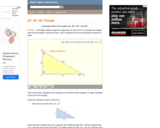Rating
0.0 stars

An interactive applet and associated web page that demonstrate the properties of a 30-60-90 triangle. The applet shows a right triangle that can be resized by dragging any vertex. As it is dragged, the remaining vertices change so that the triangle's angles remain 30 degrees, 60 degrees and 90 degrees The text on the page points out that the sides of a 30-60-90 triangle are always in the ratio of 1 : 2 : root 3 Applet can be enlarged to full screen size for use with a classroom projector. This resource is a component of the Math Open Reference Interactive Geometry textbook project at http://www.mathopenref.com.

Subject:
Math
Material Type:
Simulation
Provider:
Math Open Reference
Author:
John Page
05/15/2018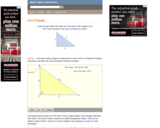Rating
0.0 stars

An interactive applet and associated web page that demonstrate the properties of a 3:4:5 triangle - one of the Pythagorean triples. The applet shows a right triangle that can be resized by dragging any vertex. As it is dragged, the remaining vertices change so that the triangle's side remain in the ration 3:4:5. The text on the page has an example of how the triangle can be used to measure a right angle on even large objects. Applet can be enlarged to full screen size for use with a classroom projector. This resource is a component of the Math Open Reference Interactive Geometry textbook project at http://www.mathopenref.com.

Subject:
Math
Material Type:
Simulation
Provider:
Math Open Reference
Author:
John Page
05/15/2018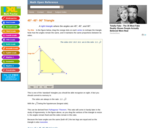Rating
0.0 stars

An interactive applet and associated web page that demonstrate the properties of a 45-45-90 isosceles right triangle. The applet shows a right triangle that can be resized by dragging any vertex. As it is dragged, the remaining vertices change so that the triangle's angles remain 45 degrees, 45 degrees and 90 degrees The text on the page points out that the sides of a 45-45-90 triangle are always in the ratio of 1 : 2 : root 2 Applet can be enlarged to full screen size for use with a classroom projector. This resource is a component of the Math Open Reference Interactive Geometry textbook project at http://www.mathopenref.com.

Subject:
Math
Material Type:
Simulation
Provider:
Math Open Reference
Author:
John Page
05/15/2018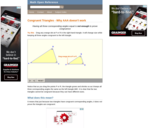Rating
0.0 stars

An interactive applet and associated web page that shows that angle-angle-angle (AAA) is not enough to prove congruence. The applet shows two triangles, one of which can be dragged to resize it, showing that although they have the same angles they are not the same size and thus not congruent. The web page describes all this and has links to other related pages. Applet can be enlarged to full screen size for use with a classroom projector. This resource is a component of the Math Open Reference Interactive Geometry textbook project at http://www.mathopenref.com.

Subject:
Math
Material Type:
Simulation
Provider:
Math Open Reference
Author:
John Page
05/15/2018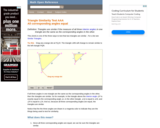Rating
0.0 stars

An interactive applet and associated web page showing how the AAA similarity test works. Two similar triangles are shown that can be resized by dragging. The other triangle adjusts to remain similar and the angle-angle-angle elements are highlighted to show how they are involved in this test of similarity. (all three interior angles congruent). The web page describes all this and has links to other related pages. Applet can be enlarged to full screen size for use with a classroom projector. This resource is a component of the Math Open Reference interactive geometry reference book project at http://www.mathopenref.com.

Subject:
Math
Material Type:
Simulation
Provider:
Math Open Reference
Author:
John Page
05/15/2018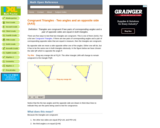Rating
0.0 stars

An interactive applet and associated web page that shows how triangles that have two angles and a non-included side the same must be congruent. The applet shows two triangles, one of which can be reshaped by dragging any vertex. The other changes to remain congruent to it and the two angles and non-included side are outlined in bold to show they are the same measure and are the elements being used to prove congruence. The web page describes all this and has links to other related pages. Applet can be enlarged to full screen size for use with a classroom projector. This resource is a component of the Math Open Reference Interactive Geometry textbook project at http://www.mathopenref.com.

Subject:
Math
Material Type:
Simulation
Provider:
Math Open Reference
Author:
John Page
05/15/2018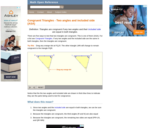Rating
0.0 stars

An interactive applet and associated web page that shows how triangles that have two angles and their included side the same must be congruent. The applet shows two triangles, one of which can be reshaped by dragging any vertex. The other changes to remain congruent to it and the two angles and the included side are outlined in bold to show they are the same measure and are the elements being used to prove congruence. The web page describes all this and has links to other related pages. Applet can be enlarged to full screen size for use with a classroom projector. This resource is a component of the Math Open Reference Interactive Geometry textbook project at http://www.mathopenref.com.

Subject:
Math
Material Type:
Simulation
Provider:
Math Open Reference
Author:
John Page
05/15/2018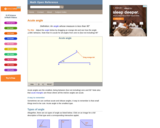Rating
0.0 stars

An interactive applet and associated web page that demonstrate acute angles (those less than 90 deg). The applet presents an angle (initially acute) that the user can adjust by dragging the end points of the line segments forming the angle. As it changes it shows the angle measure and a message that indicate which type of angle it is. There a software 'detents' that make it easy capture exact angles such as 90 degrees and 180 degrees The message and angle measures can be turned off to facilitate classroom discussion. The text on the page has links to other pages defining each angle type in depth. Applet can be enlarged to full screen size for use with a classroom projector. This resource is a component of the Math Open Reference Interactive Geometry textbook project at http://www.mathopenref.com.

Subject:
Math
Material Type:
Simulation
Provider:
Math Open Reference
Author:
John Page
05/15/2018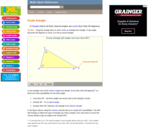Rating
0.0 stars

An interactive applet and associated web page that demonstrate the three types of triangle: acute, obtuse and right. The applet shows a triangle that is initially acute (all angles less then 90 degrees) which the user can reshape by dragging any vertex. There is a message changes in real time while the triangle is being dragged that tells if the triangle is an acute, right or obtuse triangle and gives the reason why. By experimenting with the triangle student can develop an intuitive sense of the difference between these three classes of triangle. Applet can be enlarged to full screen size for use with a classroom projector. This resource is a component of the Math Open Reference Interactive Geometry textbook project at http://www.mathopenref.com.

Subject:
Math
Material Type:
Simulation
Provider:
Math Open Reference
Author:
John Page
05/15/2018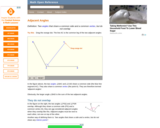Rating
0.0 stars

An interactive applet and associated web page that show the concept of adjacent angles (two angles that share a common leg). The applet shows three line segments with a common endpoint. The user can move the center one and see that the angles on both sides (the adjacent angles) of it are affected. Applet can be enlarged to full screen size for use with a classroom projector. After use in the classroom, students can access it again from any web browser at home or in the library with no login required. This resource is a component of the Math Open Reference Interactive Geometry textbook project at http://www.mathopenref.com.

Subject:
Math
Material Type:
Simulation
Provider:
Math Open Reference
Author:
John Page
05/15/2018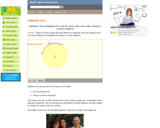Rating
0.0 stars

An interactive applet and associated web page that demonstrate the the concept of adjacent arcs. The user can 3 drag points around a circle that define the endpoints of two adjacent arcs. Web page has the properties of adjacent arcs. Applet can be enlarged to full screen size for use with a classroom projector. This resource is a component of the Math Open Reference Interactive Geometry textbook project at http://www.mathopenref.com.

Subject:
Math
Material Type:
Simulation
Provider:
Math Open Reference
Author:
John Page
05/15/2018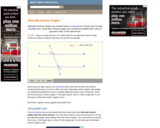Rating
0.0 stars

An interactive applet and associated web page that demonstrate the alternate exterior angles that are formed where a transversal crosses two lines. The applets shows the two possible pairs of angles alternating when in animation mode. By dragging the three lines, it can be seen that the angles are congruent only when the lines are parallel. When not in animated mode, there is a button that alternates the two pairs of angles. The text on the page discusses the properties of the angle pairs both in the parallel and non-parallel cases. Applet can be enlarged to full screen size for use with a classroom projector. This resource is a component of the Math Open Reference Interactive Geometry textbook project at http://www.mathopenref.com.

Subject:
Math
Material Type:
Simulation
Provider:
Math Open Reference
Author:
John Page
05/15/2018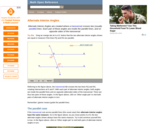Rating
0.0 stars

An interactive applet and associated web page that demonstrate the alternate interior angles that are formed where a transversal crosses two lines. The applets shows the two possible pairs of angles alternating when in animation mode. By dragging the three lines, it can be seen that the angles are congruent only when the lines are parallel. When not in animated mode, there is a button that alternates the two pairs of angles. The text on the page discusses the properties of the angle pairs both in the parallel and non-parallel cases. Applet can be enlarged to full screen size for use with a classroom projector. This resource is a component of the Math Open Reference Interactive Geometry textbook project at http://www.mathopenref.com.

Subject:
Math
Material Type:
Simulation
Provider:
Math Open Reference
Author:
John Page
05/15/2018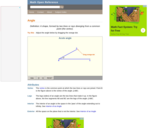Rating
0.0 stars

An interactive applet and associated web page that introduce the concept of an angle. An angle made from two line segments is shown that the user can adjust by dragging the end points of the segments. In real time, as the angles is changed by the user, the angle measure in degrees is shown and a message telling what type of angle it currently is: acute, right, obtuse, reflex or straight. Applet can be enlarged to full screen size for use with a classroom projector. This resource is a component of the Math Open Reference Interactive Geometry textbook project at http://www.mathopenref.com.

Subject:
Math
Material Type:
Simulation
Provider:
Math Open Reference
Author:
John Page
05/15/2018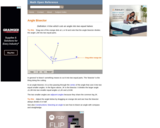Rating
0.0 stars

An interactive applet and associated web page that demonstrate the bisector of an angle. An angle is shown using two line segments that can be dragged to change the angle measure. The angle is bisected by a line which moves while dragging to always divide the angle into two equal angles. The angle measures can be turned off for class discussions. Applet can be enlarged to full screen size for use with a classroom projector. This resource is a component of the Math Open Reference Interactive Geometry textbook project at http://www.mathopenref.com.

Subject:
Math
Material Type:
Simulation
Provider:
Math Open Reference
Author:
John Page
05/15/2018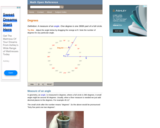Rating
0.0 stars

An interactive applet that acts as a 'digital manipulative' for explaining angles measured in degrees. The applet has an angle formed from two segment that can be dragged around in a circle. The angle measure is shown against a 'clock face' calibrated in degrees. The measures can be turned off for class angle estimation discussions. Applet can be enlarged to full screen size for use with a classroom projector. This resource is a component of the Math Open Reference Interactive Geometry textbook project at http://www.mathopenref.com.

Subject:
Math
Material Type:
Simulation
Provider:
Math Open Reference
Author:
John Page
05/15/2018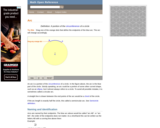Rating
0.0 stars

An interactive applet and associated web page that demonstrate the concept of an arc. The applet shows a circle with part of it highlighted to identify the arc. Each endpoint of the arc can be dragged to resize it. The web page has definitions and links to the properties of an arc. Applet can be enlarged to full screen size for use with a classroom projector. This resource is a component of the Math Open Reference Interactive Geometry textbook project at http://www.mathopenref.com.

Subject:
Math
Material Type:
Simulation
Provider:
Math Open Reference
Author:
John Page
05/15/2018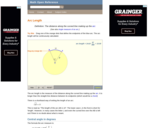Rating
0.0 stars

An interactive applet and associated web page that demonstrate the concept of arc length. The applet shows a circle with part of its circumference highlighted and the central angle shown. As the user drags either end of the arc it is redrawn and the calculation for arc length changes as you drag. The text on the web page gives the formula for calculating the arc length. Applet can be enlarged to full screen size for use with a classroom projector. This resource is a component of the Math Open Reference Interactive Geometry textbook project at http://www.mathopenref.com.

Subject:
Math
Material Type:
Simulation
Provider:
Math Open Reference
Author:
John Page
05/15/2018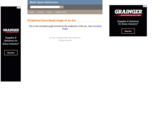Rating
0.0 stars

An interactive applet and associated web page that demonstrate the concept of the peripheral angle (inscribed angle) of an arc. This is the angle subtended at the circle circumference of an arc. The applet shows an arc with draggable end points and the angle it subtends at the circumference. It is seen that this angle is constant for a constant arc length. It shows also what happens when the vertex is inside the arc that subtends it The web page has links to other arc properties and a formula for the subtended angle for a given arc length. Applet can be enlarged to full screen size for use with a classroom projector. This resource is a component of the Math Open Reference Interactive Geometry textbook project at http://www.mathopenref.com.

Subject:
Math
Material Type:
Simulation
Provider:
Math Open Reference
Author:
John Page
05/15/2018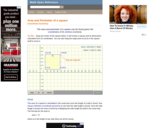Rating
0.0 stars

An interactive applet and associated web page showing how to find the area and perimeter of a square from the coordinates of its vertices. The square can be either parallel to the axes or rotated. The grid and coordinates can be turned on and off. The area and perimeter calculation can be turned off to permit class exercises and then turned back on the verify the answers. The applet can be printed as it appears on the screen to make handouts. The web page has a full description of the method for determining area and perimeter, a worked example and has links to other pages relating to coordinate geometry. Applet can be enlarged to full screen size for use with a classroom projector. This resource is a component of the Math Open Reference Interactive Geometry textbook project at http://www.mathopenref.com.

Subject:
Math
Material Type: